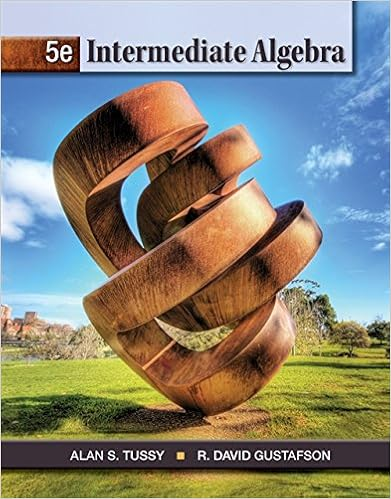# Parametric Form - Chapter 1 Integration Techniques 1.1...

• Notes
• jweng1292
• 49

This preview shows page 1 - 4 out of 49 pages.

##### We have textbook solutions for you!
The document you are viewing contains questions related to this textbook.The document you are viewing contains questions related to this textbook.
Chapter 6 / Exercise 48
Intermediate Algebra
Tussy/GustafsonExpert Verified
Chapter 1Integration Techniques1.1Integration TechniquesThe Riemann integral is defined to beZbaf(x)dx=limkΔxk→0nXi=0f(c0)(xi+1-xi)where thexipartition (a, b).However the Fundamental Theorem of Calculus allows integrals to be calculated byZbaf(x)dx=F(b)-F(a)wheredF(x)dx=f(x).This technique of finding an anti-derivative works well if an anti-derivative exists and can befound. Consider the following problems.1. Integrate the following by finding an anti-derivative.Rsin(x2)2x dx2. Why will the technique for finding the anti-derivative above not work on this integral? Note this function isintegrable.RxexdxBecause there are no simple rules for finding all anti-derivatives many techniques must be learned.1.2Integration by Parts1.2.1DerivationConsider the following use of the derivative product property and be prepared to explain the steps.f(x)=g(x)h(x).f0(x)=g(x)h0(x) +g0(x)h(x).Zf0(x)dx=Zg(x)h0(x) +g0(x)h(x)dx.g(x)h(x)=Zg(x)h0(x)dx+Zg0(x)h(x)dx.Zg(x)h0(x)dx=g(x)h(x)-Zg0(x)h(x)dx.Zudv=uv-Zv du.1
##### We have textbook solutions for you!
The document you are viewing contains questions related to this textbook.The document you are viewing contains questions related to this textbook.
Chapter 6 / Exercise 48
Intermediate Algebra
Tussy/GustafsonExpert Verified
2CHAPTER 1.INTEGRATION TECHNIQUES1.2.2ExampleConsider the following technique calledintegration by parts. RememberEquation 1Rudv=uv-Rvdu.ZxexdxZxexdx=Zxexdxu=xdv=exdxdu=dxv=ex=xex-Zexdx=xex-ex+C.1.2.3PracticeUse integration by parts to find the following anti-derivatives.1.Zxsinx dx2.Zx2sinx dx3.Zxlnx dx4.Zlnx dx5. Complete the WebAssign problems.1.2.4ExampleThe practice problems required using integration by parts in different ways. The following examples demonstratesanother use of this technique.Zexsinx dx=Zexsinx dxu=exdv=sinx dxdu=exdxv=-cosx=ex(-cosx)-Z-cosxexdx=-excosx+Zexcosx dxu=exdv=cosx dxdu=exdxv=sinx=-excosx+exsinx-Zsinxexdx=-excosx+exsinx-Zexsinx dx.
1.3.INTEGRATION OF TRIGONOMETRIC FUNCTIONS3Compare the remaining integral on the right to the original integral on the left of the equation.Zexsinx dx=-excosx+exsinx-Zexsinx dx.Glob=Zexsinx dx.Glob=-excosx+exsinx-Glob.2Glob=-excosx+exsinx.Glob=12(-excosx+exsinx).Zexsinx dx=12(-excosx+exsinx) +C.1.2.5PracticeFind the following anti-derivatives. Try using integration by parts and another method.1.Zexsinx dx2.Zexsinhx dx3. How many types of problems like this exist?4. Complete the WebAssign problems.1.3Integration of Trigonometric Functions1.3.1MotivationIntegrateRsin2ycosy dyIntegrateRcos57θsinθ dθ.Note that both were easy to integrate using a u-substitution.Note thatRsin2ycos3y dycannot be directly integrated using a u-substitution.Identities, however, can be used to convert such products of trigonometric functions into a u-substitution form.
•••